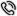服务热线4000-186-360

# RSA 算法中的计算问题

RSA 中的加密和解密过程都为求一个整数的整数次幂。如果按其含义直接计算, 则中间结果运算量非常大, 运算速度慢, 且有可能超出计算机所允许的整数取值范围。如果利用模运算性质: ( a* b) mod n= [ (a mod n) * (b mod n) ] modn, 就可以减小中间结果, 提高运算速度。

c= 0;d= 1
for ( i= k; i< = 0; i- - )
{ c= 2* c;
d= (d* d) % n;
if ( bi= = 1)

c= c+ 1;
d= ( d* a) % n;
} }
return d